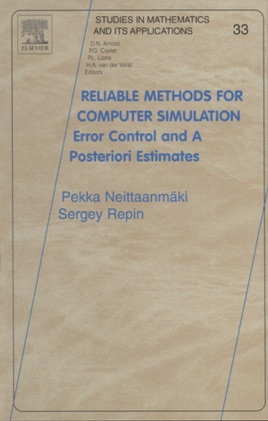• \$144.99

## Publisher Description

Recent decades have seen a very rapid success in developing numerical methods based on explicit control over approximation errors. It may be said that nowadays a new direction is forming in numerical analysis, the main goal of which is to develop methods ofreliable computations. In general, a reliable numerical method must solve two basic problems: (a) generate a sequence of approximations that converges to a solution and (b) verify the accuracy of these approximations. A computer code for such a method must consist of two respective blocks: solver and checker.

In this book, we are chiefly concerned with the problem (b) and try to present the main approaches developed for a posteriori error estimation in various problems.

The authors try to retain a rigorous mathematical style, however, proofs are constructive whenever possible and additional mathematical knowledge is presented when necessary. The book contains a number of new mathematical results and lists a posteriori error estimation methods that have been developed in the very recent time.

· computable bounds of approximation errors
· checking algorithms
· iteration processes
· finite element methods
· elliptic type problems
· nonlinear variational problems
· variational inequalities